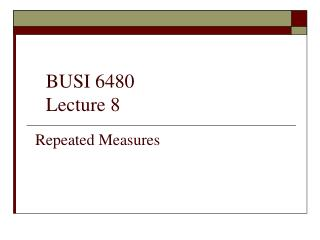DownloadDownload PresentationRepeated Measures

# Repeated Measures

Download Presentation## Repeated Measures

- - - - - - - - - - - - - - - - - - - - - - - - - - - E N D - - - - - - - - - - - - - - - - - - - - - - - - - - -
##### Presentation Transcript

1. BUSI 6480 Lecture 8 Repeated Measures

2. Repeated Measures Terminology Subject An individual or experimental unit from which a sequence of responses are recorded. Within-subjects factor: A set of conditions in which a dependent variable is measured repeatedly for all subjects. Trials: The sequential conditions of the within-subjects factor. Between-subjects factor: A set of conditions in which a dependent variable is measured on independent groups of sample members, where each group is exposed to a different condition. The conditions that constitute this factor type are called groups. Repeated measures ANOVA with between-subjects factors: An experimental design in which an analysis has both within-subjects factors and between subjects factors.

3. Repeated Measures Data: data collected over sequential time periods per subject. • Data typically take one of two formats: • A record is created for each subject with responses over time periods and independent variables. • 2. A record is created for each time period and for each subject. Thus one subject would have multiple records.

4. Typically, a Between Subject Factor and a Within Subjects Factor Are Present • Types of Program – Between Subjects • Sequence (time) – Within Subjects

5. Within Subject Covariance Structures Examples

6. Some Popular Choices for Covariance Structures VC = variance components CS = compound symmetry UN = Unstructured

7. Some Correlation/Covariance Patterns

8. Covariance Patterns – Subpatterns • Note that VC is a special case of CS. (nested) • And CS is a special case of HF. (nested) • And HF is a special case of UN. (nested) • Note: Choice of Covariance Pattern does not affect test of Between Subjects Factor.

9. GLM Repeated Measures Procedure in SAS/SPSS • Assumes either a Type H or unstructured within-subject covariance matrix • Automatically performs a sphericity test with the PRINTE option • Requires data to be inputted with one record per subject. • Estimates covariance parameters using a method of moments. • Requires balanced data – ignores subjects with missing data

10. Mixed Procedure in SAS/SPSSNote the advantages in the Mixed Approach Proc Mixed 1. Allows a wide variety of within-subject covariance structures with Type H being only one. 2. Sphericity test can be performed by running both TYPE=UN and TYPE=HF and subtracting the chi-square values and taking a difference in the degrees of of freedom. 3. Requires column format with one observation per time period. 4. Estimates covariance parameters using a (restricted) maximum likelihood method (same as used in SEM). 5. Allows data to be missing.

11. SPSS: GLM > Repeated Measures

12. SPSS: Mixed Models > Linear (not covered)

13. GLM Approach to Repeated Measures

14. Mixed Approach to Repeated Measures

15. SPSS: Define Repeated Measures -GLM

16. SPSS: GLM - Within and Between Subjects Factors

17. In SPSS, AIC and BIC (aka SBC) will appear in a box labeled Information Criteria -2LL

18. Using the Chi-Square Difference Approach for Nested Models

19. SPSS Mixed Model Dialog Box – Select Covariance Structure

20. Insert Factors, Covariates, and Residual Weight and Dependent Variable

21. Example of Fixed Effects Model Building Dialog Box.Always include Intercept (unless you have a reason not to)

22. Under Subject Groupings, the variable Worker can be specified, unless only a Fixed Effects model is used.

23. Tests for Covariance Parameters and Parameter Estimates are Options under Statistics

24. SPSSSelect Restricted Max Likelihoodfor most mixed models. Increase max iterations for ill conditioned data.

25. Trends in Repeated Measures In SPSS GLM, click on contrast within Repeated Measures and select polynomial. In Mixed in SPSS, add additionally created square and cube terms and interactions with these terms if necessary to data set. When using a polynomial in the repeated measure, the variable Day, its square and cubic terms are used as covariates and Day is not a factor variable. The notation for an interaction between a repeated variable and a between groups factor may be expressed as the between-subjects factor listed with the repeated variable next to it in parenthesis. The degrees of freedom for random effects can be fractional rather than integer values, indicating that fractions of sources of variation were used in synthesizing appropriate error terms.

26. Example in Getting p-value for Chi-square Statistic • Data mypvalue; • x=18.307; df = 10; • pvalue = 1- probchi(x,df); • proc print data=mypvalue; • run; • quit; • Solution is a p-value of .05 in print out.

27. Repeated Measures Experiments Often Have Missing Values for Several Time Periods • SAS • proc mi data=MyData round=.1 seed=5432 nimpute=1 out= outmi; • var myDepVar myTimePeriod1-myTimePeriod4; • run; • proc print data = outmi; NOTE: SAS uses a Markov Chain Monte Carlo method to estimate missing values in Proc mi.

28. Examine Output of Missing Data Patterns (patterns should be random) • 1 X X X X X X X 39 • 2 X X X X X . X 4 • 3 X X X X . X X 3 • 4 X X . X X X X 7 • 5 X . X X X X X 6 • 6 X . X X . X X 1 Ideally, no one pattern should have more than 25% of the missing values (except for the pattern with no missing values).